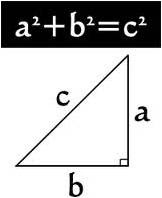## Sunday, January 22, 2012

### TIME = SPACE SERIES. PART 17 – SEELAM - THE PRIMORDIAL RHYTHM & FUNDAMENTALS

Now, let us get into some real heavy stuff - Mathematics (Ganitham – the study of Ganas) that governs this rhythm and harmonics. My first concern is how to make it easily digestible for an average individual who hates Maths? So I shall take the easiest route that I know. I shall first explain the concept, then the actual Maths part of it and then go to the philosophy and significance of the same. Later we shall look at how these are codified in nature and our scriptures and available in front of our eyes which we have failed to recognize.

Let us be clear about the following terminologies like Progression, Proportion, root, Diagonal and Pythagoras theorem:

·         Let us take a series as follows: 2, 4, 8, 16, 32…. In this we need to be clear about what is Progression and proportion. As many of you know that whole series is a geometric progression where the next number in the series is a function of the current number and a multiple. This multiple here is 2, so that 2*2 = 4, 4*2 = 8 and so on. This multiple or the constant is called as proportion. You derive the value of the proportion by dividing any number by its previous number – (n+1)/n : (n+2)/(n+1). Hope this is clear.

·         We know that a square has 4 equal sides. Each side of a square is called as “root” and the line that connects the 2 opposite ends is called as ‘Diagonal”. In the figure below sides AB, BC, CD, DA are called as “Root”. Lines AC, BD are called as ‘Diagonal”.Square                                                                             Pythagoras theorem

·         Pythagoras theorem – This theorem gave us the formula to calculate the sides of a right angle triangle as given above. If you are not familiar with this, then please understand that if you know any 2 sides of the triangle the 3rd side can be calculated by the formula given above. I am not going to focus on the argument that this theorem was available in Indian scriptures before the Greek mathematician gave it. Let’s look at the concepts and not which civilization gets credit as of now.

Since the fundamental structure is a square in 2D and Cube in 3D and also in the process of evolution one becomes many, let’s ask ourselves the following questions:

·       How one square does create another square which is larger in size, progressively? What is the progression and proportion in this series?
·   How one cube does create shapes which are polygonal (Hexagon, Octagon, decagon, dodecagon etc) progressively? What is the progression and proportion in this series?

Now let us see the diagram as below where and consider the square ABGF. You can enlarge the image to see the alphabets clearly. Now as we know sides AB, BG, GF, AF are called “root” – remember this well and let us assume the smallest root possible is unity and hence each root is of unit length. AB=BG=GF=AF=1. Area of this square = 1*1 = 1.

Line AG is called as the “diagonal” and as per Pythagoras theorem the length of AG should be √2AG = √(AF2+GF2). So to summarize the root of the square ABGF is 1 and the diagnol is √2.

Now consider a larger square AGHJ, here AG, GH, HJ, AJ are the sides and hence called as root. AH, GJ are the diagonal in the 2nd square. We know AG = √2, then AH = GJ = 2 as per Pythagoras theorem. To sum up the root of the square AGHJ is √2 and the diagonal is 2. Area of this square is 2.

If you look at the ratio root/diagonal (root to diagonal ratio) of both the squares it is 1/√2 and √2/2 which results in 1/√2. This will progressively hold good. The magic proportion in this progression is √2.

What we can infer from this is if we consider a progression of squares from a unit square to larger squares, then the proportion of the progression is √2. But before we jump into its philosophical significance let’s answer the second question.

Consider the cube above whose side is of unit length. We know a cube is made of squares. We know ABCD is a square of length (root) =1 and diagonal = AC = √2. If we want to find out the length of the diagonal of the cube which is AE, then ACE is a right angle triangle with AC = √2. And CE =1 and hence AE = √3.

What we can infer from this is if we consider a progression of cubes from a unit cube to larger ones, then the proportion of the progression is √3.

I am going to handle the significance of √2 and √3 in the next part.

Happy reading!